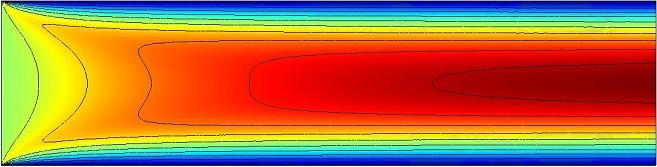# Stem flow - scaling solutionOnce water crosses from xylem to phloem, it flows through the trunk toward the energy consuming parts of the tree. The water becomes solvent for sucrose, and the flowing material in phloem is sap. The phloem is essentially one long pipe of constant radius, with length equal to the height of the tree.

Compared to water, sap is significantly more viscous and thus will exert friction on itself that slows its flow. As with the membrane, we can find a relation between osmotic pressure and volume flow that we call the hydraulic resistance of the sap in the stem, $\mathcal{R}_{\text{stem}}$.

We can calculate this resistance exactly but it is a little involved, so we'll first try to derive its functional form by scaling arguments. As the great John Wheeler once said, "Never make a calculation until you know the answer."

We expect the area of the cross section, the length of the pipe $h$, the pressure across the pipe $\Delta p$, and the viscosity of the liquid $\eta$ to come into play.

Use physical intuition and dimensional analysis to come up with a relation, $\displaystyle u \sim f(A,\eta,\Delta p,h)$ between flow rate $u$, viscosity $\eta$, pipe length $h$, cross sectional area $A$, and pressure $\Delta p$. Use limiting cases to check your hypothesis.


Details and Assumptions:

• The units of viscosity, $\eta$, are Pascal seconds.
×